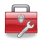# Using Statistical Regression Methods in Education Research# 4.13 Evaluating Interaction Effects

Interaction terms

We saw in Module 3when modelling a continuous measure of exam achievement (the age 14 average test score) that there were significant interactions between ethnic group and SEC (if you want to remind yourself about interaction effects head to Page 3.11). There are therefore strong grounds to explore whether there are interaction effects for our measure of exam achievement at age 16.

The first step is to add all the interaction terms, starting with the highest. With three explanatory variables there is the possibility of a 3-way interaction (ethnic * gender * SEC). If we include a higher order (3 way) interaction we must also include all the possible 2-way interactions that underlie it (and of course the main effects). There are three 2-way interactions: ethnic*gender, ethnic*SEC and Gender*SEC. Our strategy here is to start with the most complex 3-way interaction to see if it is significant. If it is not then we can eliminate it and just test the 2-way interactions. If any of these are not significant then we can eliminate them. In this way we can see if any interaction terms make a statistically significant contribution to the interpretation of the model.

In this example we will use the MLR LSYPE 15,000dataset because it contains some useful extra variables which we created for the last module. The process for creating a model with interaction terms is very similar to doing it without them so we won’t repeat the whole process in detail (see the previous page, Page 4.12, if you require a recap). However, there is a key extra step which we describe below...

Entering interaction terms to a logistic model

The masters of SPSS smile upon us, for adding interaction terms to a logistic regression model is remarkably easy in comparison to adding them to a multiple linear regression one! Circled in the image below is a button which is essentially the ‘interaction’ button and is marked as ‘>a*b>’. How very helpful! All you have to do is highlight the two (or more) variables you wish to create an interaction term for in the left hand window (hold down ‘control’ on your keyboard while selecting your variables to highlight more than one) and then use the ‘>a*b>’ button to move them across to the right hand window as an interaction term.

The two variables will appear next to each other separated by a ‘*’. In this way you can add all the interaction terms to your model.Reducing the complexity of the model

If we were to create interaction terms involving all levels of SEC we would probably become overwhelmed by the sheer number of variables in our model. For the two-way interaction between ethnicity and SEC alone we would have seven ethnic dummy variables multiplied by seven SEC dummy variables giving us a total of 49 interaction terms! Of course, we could simplify the model if we treated SEC as a continuous variable, we would then have only seven terms for the interaction between ethnic * SEC. While it would be a more parsimonious model (because it has fewer parameters to model the interaction), treating SEC as a continuous variable would mean omitting the nearly 3,000 cases where SEC was missing. The solution we have taken to this problem, as described before on Page 3.12, is to use the shortened version of the SEC variable called SECshort which has only three (rather than eight) SEC categories (plus a code for missing values). That should make our lives a little less confusing!

Even though we have chosen to use the three category SEC measure, the output is very extensive when we include all possible interaction terms. We have a total of 55 interaction terms (three for gender*SECshort, seven for ethnic*gender, 21 for ethnic*SECshort and a further 21 for ethnic*SECshort*gender). You will forgive us then if we do not ask you to run the analysis with all the interactions! Instead we will give you a brief summary of the preliminary analyses, before asking you to run a slightly less complex model. Our first model included all the three-way and two way interactions as well as the main effects. It established that three-way interaction was not significant (p=0.91) and so could be eliminated. Our second model then included just all the two-way interactions (and main effects). This showed that the gender*SECshort and the ethnic*gender interactions were also not significant but the ethnic*SECshort interaction was significant. The final model therefore eliminated all but the ethnic*SECshort interaction which needs to be included along with the main effects.

Running the logistic model with an interaction term

So let’s run this final model including the ethnic*SECshort interaction. Maybe you want to run through this example with us on SPSS (you can also follow it in our video demonstration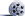). In this model the ‘dependent’ variable is fiveem (our outcome variable) and the ‘covariates’ (our explanatory variables) are ethnic, gender, SECshort, and ethnic*SECshort (the interaction term, which is entered in the way that we showed you earlier on this page). Your final list of variables should look like the one below.Remember to tell SPSS which variables are categorical and set the options as we showed you on Page 4.11!

Before running this model you will need to do one more thing. Wherever it was not possible to estimate the SEC of the household in which the student lived SECshort was coded 0. To exclude these cases from any analysis the ‘missing value’ indicator for SECshort is currently set to the value ‘0’. As discussed on Page 3.9, it is actually very useful to include a dummy variable for missing data where possible. If we want to include these cases we will need to tell SPSS. Go to the ‘Variable view’ and find the row of options for SECshort. Click on the box for Missing and change the option to ‘No missing values’ (see below) and click OK to confirm the change.This will ensure that SPSS makes us a dummy variable for SEC missing. You can now click OK on the main menu screen to run the model!

Interpreting the output

The results of this final model are shown below. Rather than show you all of the output as on the previous page (Page 4.12), this time we will only show you the ‘Variables in the Equation’ table (Figure 4.13.1) as it is most relevant to interpreting interaction effects.

Figure 4.13.1: Variables in the Equation Table with Interaction TermsThe overall Wald for the SECshort*ethnic interaction is significant (WALD=43.8, df=21, p<.005) so we proceed to look at the individual regression coefficients. You will need to refer to the Categorical Variables Encoding Table to remind yourself what each of these coefficients represents (for example SECshort(1)=missing; SECshort(2)= high SEC and SECshort(3)= middle SEC, with low SEC as the reference category). There are statistically significant coefficients for the interaction between Pakistani and middle and high SEC, between Bangladeshi and middle and high SEC and between Black Caribbean and high SEC.

Working out the Odds Ratios (ORs) with interaction effects is somewhat tricky (remember we encountered a similar issue for multiple linear regression modules on Page 3.11). As we have discussed, each B coefficient represents the change in the logit of our outcome predicted by a one unit change in our explanatory variable, but this is more complicated when we are also have interactions between our explanatory variables.

• Each of the ethnic coefficients represents the difference between that ethnic group and ‘White British’ students, but crucially only for students in the baseline category for SECshort (i.e. low SEC students).
• For SECshort the coefficients represent the difference between each of the medium and high SEC categories and the baseline category of low SEC, but only for ‘’White British’ students.
• The coefficients for each ethnic * SECshort interaction term represent how much the SEC contrasts vary for each ethnic group, relative to the size of the SEC effect among White British students.

Interpreting the SEC gap for different ethnic groups

We can see that SEC has a substantial association with achievement among White British students. White British students from high SEC homes are 5.67 times more likely to achieve fiveem than White British students from low SEC homes. How do we determine the size of the SEC effect among other ethnic groups? Well, when interaction terms are included in the model we need to calculate the predicted probabilities by adding the B coefficients together, as we did in multiple linear regression. So to estimate the SEC gap for Black Caribbean students we add the coefficient for high SEC [SECshort(2)] and the B for the interaction between Black Caribbean and high SEC [SECshort(2) by ethnic(5)]. This gives = 1.735 + -.784 = 0.953. What does this mean? Not much yet – we need to take the exponent to turn it into a trusty odds ratio: Exp(0.953)=2.59 (we used the =EXP() function in EXCEL or you could use a calculator). This means that among Black Caribbean students High SEC students are only 2.6 times more likely to achieve fiveem than low SEC students - the SEC gap is much smaller than among White British students. The important point to remember is that you cannot simply add up the Exp(B)s to arrive here – it only works if you add the B coefficients in their original form and then take the exponent of this sum!

Interpreting the ethnic gaps at different levels of SEC

The model has told us what the ethnic gaps are among low SEC students (the reference category). Suppose I wanted to know what the estimated size of the ethic gaps was among high SEC students, how would I do this? To find out you would rerun the model but set high SEC as the base or reference category. The coefficients for each ethnic group would then represent the differences between the average for that ethnic group and White British students among those from high SEC homes.

Currently SECshort is coded as follows with the last category used as the reference group.

 SECshort value Label 0 Missing SEC 1 High SEC 2 Middle SEC 3 Low SEC (Reference category LAST)

We can simply recode the value for missing cases from 0 to 9 and set the reference category to the first value, so High SEC becomes the reference category, as shown below:

 SECshort value Label 1 High SEC (Reference category FIRST) 2 Middle SEC 3 Low SEC 4 Missing SEC

You can do this through the Transformation-Recode into new variable windows menu (see Foundation Module) or simply through the following syntax:

Syntax alert! RECODE SECshort (0=4) (ELSE=Copy) INTO SECshortnew. EXECUTE

We then rerun the model simply adding SECshortnew as our SEC measure. It is important to note that computationally this is an exactly equivalent model to when low SEC was the reference category. The regression coefficients for other variables (for example, gender) are identical, the contrast between low and high SEC homes is the same (you can check the B value in the output below), and the R2 and log-likelihood are exactly the same. All that has varied is that the coefficients printed for ethnicity are now the contrasts among high SEC rather than low SEC homes.

The output is shown below (Figure 4.13.2). For convenience we have added labels to the values so you can identify the groups. As you know, this is not done by SPSS so it is vital that you refer to the Categorical variables encoding table when interpreting your output. It is apparent that the ethnic gaps are substantially different among high SEC than among low SEC students. Among low SEC students the only significant contrasts were that Indian, Bangladeshi and Any other ethnic group had higher performance than White British (see Figure 4.13.1). However among students from high SEC homes while Indian students again achieve significantly better outcomes than White British students, both Black Caribbean (OR=.36, p<.005) and Black African (OR=.685, p<.025) are significantly less likely to achieve fiveem than White British students by a considerable margin. Black Caribbean students are only about one third as likely to achieve fiveem as White British high SEC students. In percentage terms we can say they are 64% (0.358-1 * 100) less likely to achieve fiveem than White British students of the same SEC group.

Figure 4.13.2: Variables in the Equation Table with high SEC as the reference category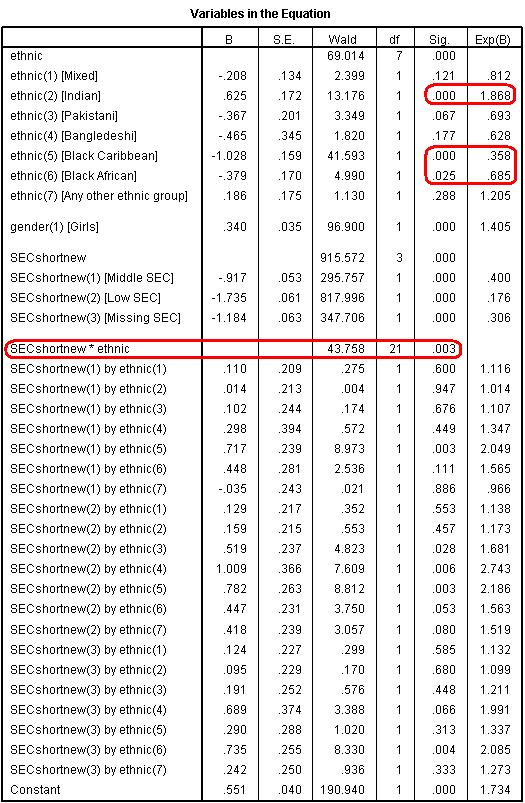If we wanted to evaluate the ethnic gaps among students from middle SEC homes we would simply follow the same logic as above, recoding our values so that middle SEC was the first (or last) category and setting the reference category appropriately.

Putting it all together - viewing the interactions graphically

What is most helpful in understanding these interactions is to plot the data graphically. This gives us an easy visual indicator to help in interpreting the regression output and the nature of any interaction effects. We have done this in Figure 4.13.3 below. You can use Graphs > Legacy Dialogs > Line to create this graph or alternatively you can use the syntax below (see the Foundation Moduleif you require further guidance). Here we have plotted the actual means for fiveem, but you could equally plot the predicted probabilities if you saved them from the model (see Page 4.11). Note that in the graph we have omitted cases where SEC is missing by returning the missing value for SECshort to ‘0’ before requesting the graph.

Syntax Alert! GRAPH /LINE(MULTIPLE)=MEAN(fiveem) BY SECshort BY ethnic.

Figure 4.13.3: Mean Number of Students with Five or More A*-C grades (inc. English and Maths) by SEC and EthnicityThe line graph shows a clear interaction between SEC and ethnicity. If the two explanatory variables did not interact we would expect all of the lines to have approximately the same slope (for example, the lines on the graph would be parallel when there is no interaction effect) but it seems that the effect of SEC on fiveem is different for different ethnic groups. For example the relationship appears to be very linear for White British students (blue line) – as the socio-economic group becomes more affluent the probability of fiveem increases. This not the case for all of the ethnic groups. For example, with regard to Black Caribbean students there is a big increase in fiveem as we move from low SEC to intermediate SEC, but a much smaller increase as we move to high SEC. As you (hopefully) can see, the line graph is a good way of visualising an interaction between two explanatory variables.

Now that we have seen how to create and interpret out logistic regression models both with and without interaction terms we must again turn our attention to the important business of checking that the assumptions underlying our model are met and that the results are not misleading due to any extreme cases.

 HomeModules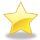Site Guide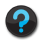Module 4 Contents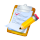Resources